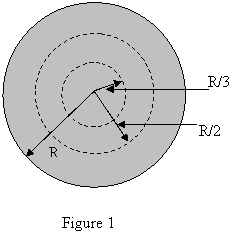KFUPM-Physics102-052
Dr.Aljalal
Sec16
Sec17
Sec18

Recitation ch 26

Question 1
A cylindrical wire of radius R = 2.0 mm has a uniform current
density J = 2.0*10**(5) A/m**2. What is the current through
the portion of the wire between radial distances R/3 and R/2?
(see figure 1)a   1.95 A.
b   3.73 A.
c   5.31 A.
d   0.35 A.
e   9.11 A.
==============================================
Question 2
A heater element of resistance 10**3 Ohm is constructed
to operate at 110 V. How much thermal energy is produced
in one hour by the heater?

a   4.4*10**4 J.
b   2.2*10**7 J.
c   5.1*10**2 J.
d   6.2*10**5 J.
e   1.9*10**5 J.
==============================================
Question 3
A current of 0.300 A is passed through a lamp for 2.00 minutes
using a 6.00-V battery. The energy dissipated by the lamp during
the 2.00 minutes is:

a   216 J
b   20.0 J
c   36.0 J
d   1.80 J
e   12.0 J
==============================================
Question 4
A copper wire of cross sectional area 3.00*10**(-6) m**2
and length 4.00 m has a current of 2.25 A uniformly
distributed across that area.
How much electric energy is converted to thermal energy
in 10.0 minutes ?
[The resistivity of copper is 1.68*10**(-8) ohm*m]

a   0.50 J
b   68.0 J
c   13.6 J
d   21.7 J
e   30.2 J
==============================================
Question 5
If 4.7*10**(16) electrons pass a particular point in a wire
every minute, what is the current in the wire?

a   2.9*10**(-5) A.
b   1.3*10**(-4) A.
c   4.7*10**(-3) A.
d   2.9*10**(-3) A.
e   9.1*10**(-3) A.
==============================================
Question 6
An electric device, which heats water by immersing a
resistance wire in the water, generates 153 J of heat per
second when an electric potential difference of 12 V is
placed across its ends. What is the resistance of the
heater wire?

a  0.81 Ohms
b  0.58 Ohms
c  0.94 Ohms
d  2.10 Ohms
e  0.48 Ohms
==============================================
Question 7
At T = 20 degrees-C, the length of a wire is 10 m, and its
cross sectional area is 0.50 mm**2. A potential difference
of 1.0 V is maintained across the ends of the wire. The
resistance of the wire changes by 0.30 ohms for a temperature
change of 50 Celsius degrees.
what is the temperature coefficient of resistivity of the wire ?
At T = 20 degrees, the resistivity is 5.0*10**(-6) ohm*m.

a   15*10**(-3) degrees**(-1)
b   15*10**(-4) degrees**(-1)
c   15*10**(-5) degrees**(-1)
d   6.0*10**(-5) degrees**(-1)
e   6.0*10**(-4) degrees**(-1)
==============================================
Question 8
Conduction electrons move to the right in a certain
wire. This indicates that:

a   the current density points to the right but
the electric field points to the left.
b   the current density and the electric field both
point to the right.
c   the current density points to the left but
the electric field points to the right.
d   the current density points to the left but
the direction of the electric field is unknown.
e   the current density and the electric field both
point to the left.
==============================================
Question 9
A metal wire of resistance R is cut into three equal
pieces that are then connected side by side to form a
new wire the length of which is equal to one-third the
original length. What is the resistance of the new wire ?
a   R
b   R/3
c   9*R
d   R/9
e   3*R
==============================================
Question 10
A copper wire of cylindrical shape has resistance R.
What is the resistance of a second wire, made of the
same material, that is twice as long and has twice the
diameter ?

a   R
b   4R
c   2R
d   R/2
e   R/4
==============================================
Question 11
A copper wire "1" has a length L1 and diameter d1. Another
copper wire "2" has a length L2 and diameter d2. At constant
temperature, the second conductor has smaller resistance if:

a  d2 = d1 and L2 > L1.
b  d2 < d1 and L2 < L1.
c  d2 < d1 and L2 = L1.
d  d2 > d1 and L2 > L1.
e  d2 > d1 and L2 < L1.
==============================================